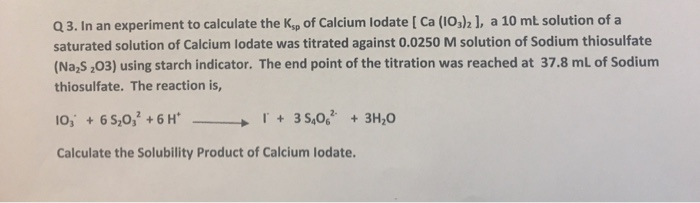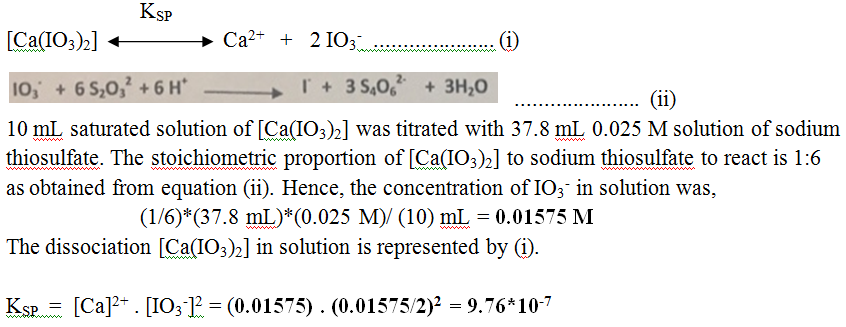# Question & Answer: In an experiment to calculate the K_sp of Calcium Iodate [Ca (IO_3)_2], a 10 mL solution of…..In an experiment to calculate the K_sp of Calcium Iodate [Ca (IO_3)_2], a 10 mL solution of a saturated solution of Calcium lodate was titrated against 0.0250 M solution of Sodium thiosulfate (Na_2 S_2 O_3) using starch indicator. The end point of the titration was reached at 37.8 mL of Sodium thiosulfate. The reaction is, IO_3^- + 6 S_2 O_3^2 + 6 H^+ rightarrow I^- + 3 S_4 O_6^2- + 3H_2 O Calculate the Solubility Product of Calcium lodate.